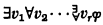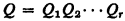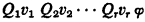Home

# The diversity of quantifier prefixes1

## Extract

The Arithmetical Hierarchy Theorem of Kleene  states that in the complete theory of the standard model of arithmetic there is for each positive integer r a Σr0 formula which is not equivalent to any Πr0 formula, and a Πr0 formula which is not equivalent to any Πr0 formula. A Πr0 formula is a formula of the formwhere φ has only bounded quantifiers; Πr0 formulas are defined dually.

The Linear Prefix Theorem in  is an analogous result for predicate logic. Consider the first order predicate logic L with identity symbol, countably many n-placed relation symbols for each n, and no constant or function symbols. A prefix is a finite sequenceof quantifier symbols ∃ and ∀, for example ∀∃∀∀∀∃. By a Q formula we mean a formula of L of the formwhere v1, …, vr are distinct variables and φ has no quantifiers. A sentence is a formula with no free variables. The Linear Prefix Theorem is as follows.

Linear Prefix Theorem. Let Q and q be two different prefixes of the same length r. Then there is a Q sentence which is not logically equivalent to any q sentence.

Moreover, for each s there is a Q formula with s free variables which is not logically equivalent to any q formula with s free variables.

For example, there is an ∀∃∀∀∀∃ sentence which is not logically equivalent to any ∀∃∃∀∀∃ sentence, and vice versa. Recall that in arithmetic two consecutive ∃'s or ∀'s can be collapsed; for instance all ∀∃∀∀∀∃ and ∀∃∃∀∀∃ formulas are logically equivalent to Π40 formulas. But the Linear Prefix Theorem shows that in predicate logic the number of quantifiers in each block, as well as the number of blocks, counts.

## Footnotes

Hide All
1

This research was supported in part by National Science Foundation grant GP-27633.

Hide All

# The diversity of quantifier prefixes1

## Metrics

### Full text viewsFull text views reflects the number of PDF downloads, PDFs sent to Google Drive, Dropbox and Kindle and HTML full text views.

Total number of HTML views: 0
Total number of PDF views: 0 *Loading metrics...

### Abstract viewsAbstract views reflect the number of visits to the article landing page.

Total abstract views: 0 *Loading metrics...

* Views captured on Cambridge Core between <date>. This data will be updated every 24 hours.

Usage data cannot currently be displayed.